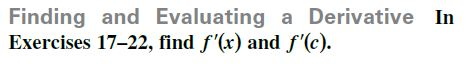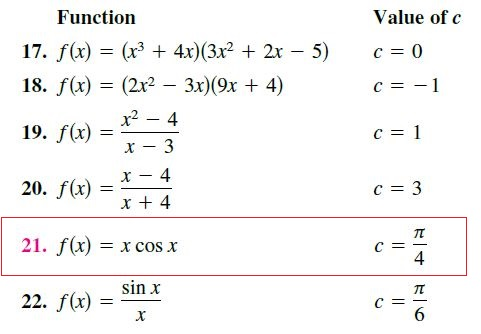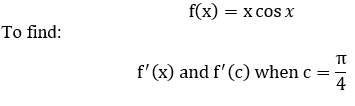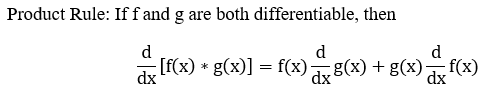# Finding and Evaluating a Derivative InExercises 17-22, find f'(x) and f'(c). FunctionValue of c17. f(x) = (x3 + 4x)(3x² + 2x5)18. f(x) = (2x2 – 3x)(9x + 4)c = -1x219. f(x)4320. f(x)21. f(x)= xCOs x4sin x22. f(x)6.х||||

Question
4 viewshelp_outlineImage TranscriptioncloseFinding and Evaluating a Derivative In Exercises 17-22, find f'(x) and f'(c). fullscreenhelp_outlineImage TranscriptioncloseFunction Value of c 17. f(x) = (x3 + 4x)(3x² + 2x 5) 18. f(x) = (2x2 – 3x)(9x + 4) c = -1 x2 19. f(x) 4 3 20. f(x) 21. f(x) = x COs x 4 sin x 22. f(x) 6. х || || fullscreen
check_circle

Step 1

Given functionStep 2

Note:...

### Want to see the full answer?

See Solution

#### Want to see this answer and more?

Solutions are written by subject experts who are available 24/7. Questions are typically answered within 1 hour.*

See Solution
*Response times may vary by subject and question.
Tagged in